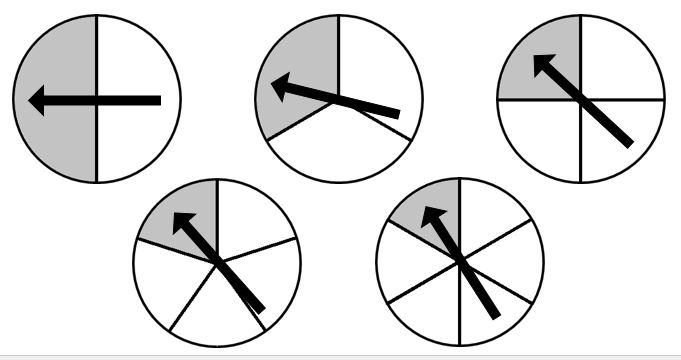# Probability with Spinners

Directions: Select three of the spinners from the image below (you may pick more than one of each) such that the total number of sectors in all three spinners totals 10. Select spinners so that the probability of all three spinners landing in the shaded sector is the smallest (or largest).

Extension: How would the answer change if you could create any number of spinners instead of exactly 3 spinners such that the total number of sectors totals 10? (e.g. 1 spinner with 10 equal sectors or 5 spinners each with 2 equal sectors)### Hint

How do you calculate the chances of two or more independent events?

How can you make the probability the smallest (or largest) number possible?

1) The greatest probability: 1/2 * 1/2 * 1/6 = 1/24
2) 1/2 * 1/3 * 1/5 = 1/30
3) 1/4 * 1/4 * 1/2 = 1/32
4) The least probability: 1/3 * 1/3 * 1/4 = 1/36

Source: Erick Lee

## Two Step Inequality with Fractional Coeffcient

Directions: Using the digits 1 to 7 at most one time each, place a digit …

1.2.